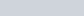# Uniform rate of the rate of change

The Merton Rule, which dates to the Middle Ages, relates a uniform change rate to its initial and final rates. Because of its main application, it is also called the Mean Speed Theorem, which in modern language states that a uniformly accelerating body over a period of time traverses the same distance as the product of the average velocity (of the initial and final velocities) with the time period:

Because the Merton Rule applied to any magnitude that changes uniformly, there was a question about its application to free fall. Galileo argued (and later observed) that free fall is a uniform rate of acceleration, and so the free fall distance is proportional to the time interval squared. In modern terms:

The question was whether free fall could also be expressed as the rate of change increasing uniformly with respect to distance. Galileo argued that it could not. Those who argued that it could failed because they continued to use velocity for their rate of change.

The simple reason that velocity does not increase uniformly with respect to distance is that uniform acceleration is defined in terms of the time interval, not the distance. To make the equation in terms of distance requires making distance the independent variable.

Let’s follow I. Bernard Cohen’s exposition of the wrong way to do it but use the right way instead. [“Galileo’s Rejection of the Possibility of Velocity Changing Uniformly with Respect to Distance” in Isis, Vol. 47, No. 3 (Sep. 1956), pp. 231-235.]

Begin with the (general) Merton Rule and that lenticity increases (or decreases) uniformly with respect to distance for some motion. Then consider a body starting from the zero location and passing through a distance D1 in a time T1. The lenticity W1 at location D1 is assumed to be proportional to the distance, so thatIf the same body were allowed to pass through a distance D2 twice the original distance D1 in a time T2, the lenticity would beFrom the Merton Rule for lenticity we have that

and

Then

and

Either way, for constantly increasing (or decreasing) lenticity the time interval is proportionate to the distance squared.